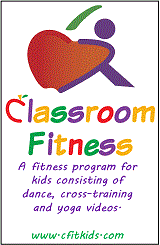Saturday, October 19 2019
Welcome GuestNumber and Number Sense
K.1 Comparing sets
K.2 Counting objects
K.3 Ordinals: 1st to 10th
K.4a Rote counting
K.4b One more (less) than a number
K.4c Count by fives and tens
K.5 Fractions-halves, fourths
Computation and Estimation
K.8 Measurement tools
K.9 Telling time
K.10 Measurement comparisons
K.11a Identify shapes
K.11b Compare size and shape
Probability and Statistics
K.13 Gather data
K.14 Graphs/tables
Patterns, Functions, and Algebra
K.15 Sort and classify
Number and Number Sense
1.1a Count/write 0 - 100
1.1b Tens & ones - place value
1.2 Skip count (2, 5, 10)
1.3 Identify/represent halves, thirds, fourths
Computation and Estimation
1.4 Reasonable magnitude
1.6 Create and solve story problems
1.7 Coin values to total of 100 cents
1.8 Time to the half hour
1.9 Nonstandard length, weight, volume
1.10a Compare volumes
1.10b Compare weights
1.11 Calendar language
1.12 Identify, describe, sort figures
1.13 Figures in the environment
Probability and Statistics
1.14 Data: tables, picture and object graphs
1.15 Interpret information from graph
Patterns, Functions, and Algebra
1.16 Sort/classify-color, size, thickness, shape
1.17 Recognize, describe, extend, create patterns
1.18 Understand equality through the use of the = sign
Number and Number Sense
2.1a Read, write, identify place value (3 digits)
2.1b Round 2-digit numbers
2.1c Compare numbers
2.2 Ordinal positions 1st to 20th, write ordinal numbers
2.3 a, b Fractions: halves, thirds, fourths, sixths, eighths
2.3c Compare unit fractions
2.4 Counting forward and backward, even/odd
Computation and Estimation
2.5 Recall addition/subtraction facts to 20
2.6 Estimate and find sums
2.7 Estimate and find differences
2.8 Solve one- and 2-step + and - problems
2.9 Related facts
2.11a Length: cm and inch
2.11c Liquid volume
2.14 Temperature
2.16 Plane and solid figures
Probability and Statistics
2.18 Use data to predict outcomes
2.19 Analyze data in graphs
Patterns, Functions, and Algebra
2.21 Solve problems by completing numerical sentences
2.22 Understand equality
Number and Number Sense
3.1a Place Value: 6 digits
3.1b Rounding: 4 digits
3.1c Comparing Numbers: 4 digits
3.2 Inverse relationships /-/x/Ã·
3.3 Fractions and mixed numbers
3.3c Compare fractions
Computation and Estimation
3.5 Multiplication and division facts
3.6 Represent multiplication/division
3.9 U.S. Customary/Metric units to measure
3.10 Area/perimeter
3.11 Telling time/elapsed time
3.12 Equivalent periods of time
3.13 Temperature
3.14 Plane/solid geometric figures
3.15 Points, lines, and angles
3.16 Congruence
Probability and Statistics
3.17 Organize data and construct graphs
3.18 Probability
Patterns, Functions, and Algebra
3.20 Properties/equality
Basic Facts Practice
Multiplication and Division
Number and Number Sense
4.1 7-digit numbers
4.3 Decimals to thousandths
Computation and Estimation
4.4a Estimation (+ -x Ã·)
4.4b Whole number + - x
4.4c Whole number computation Ã·
4.4d Solve single/multistep problems
4.5a Greatest common factor/Least common multiple
4.5d Solve problems with fractions/decimals
4.8 Liquid volume
4.9 Elapsed Time
4.10 Points, lines, line segments, rays, angles
4.11 Translation, reflection, rotation
Probability and Statistics
4.13 Probability
4.14 Line and bar graphs
Patterns, Functions, and Algebra
4.15 Numerical and geometric patterns
4.16 Equality/Associative Property
Basic Facts Practice
Multiplication and Division
Number and Number Sense
5.1 Rounding decimals
5.2 Fractions/decimals
5.3 Prime/composite & even/odd
Computation and Estimation
5.5 Computation-decimals
5.4 Whole number problems + - x Ã·
5.6 Fractions + -
5.7 Order of Operations
5.8 Perimeter, area, volume, temperature
5.10 Elapsed Time
5.11 Angle measure
5.12 Classify angles/triangles
5.13 Plane figures
Probability and Statistics
5.14 Probability-sample space
5.15 Stem & leaf plots, line graphs
5.16 Mean, median, mode, range
Patterns, Functions, and Algebra
5.18 Variables/equations
5.19 Distributive Property
Basic Facts Practice